Chart

(diff) ← Older revision | Latest revision (diff) | Newer revision → (diff)

curvilinear coordinate system, parametrization of a setA one-to-one mapping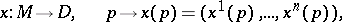of a setonto an open subsetof the real vector space. The integeris called the dimension of the chart, and the components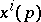of the vector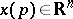are called the coordinates of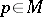with respect to the chart.

An example of a chart is the Cartesian coordinate system in the plane and in space, introduced by P. Fermat and R. Descartes, and taken by them as the basis for analytic geometry. e root','../p/p074630.htm','Series','../s/s084670.htm','Stability of an elastic system','../s/s087010.htm','Siegel disc','../s/s110120.htm','Theta-function','../t/t092600.htm','Trigonometric series','../t/t094240.htm','Two-term congruence','../t/t094620.htm','Umbral calculus','../u/u095050.htm','Variation of constants','../v/v096160.htm','Variational calculus','../v/v096190.htm','Variational calculus, numerical methods of','../v/v096210.htm','Venn diagram','../v/v096550.htm','Zeta-function','../z/z099260.htm')" style="background-color:yellow;">L. Euler was the first to employ charts (curvilinear coordinates) on surfaces in geometric research. B. Riemann took up the notion of a chart as the basis for a new infinitesimal approach to the foundations of geometry (see ). In Riemann's view, the basic object of study in geometry is a manifold — a setendowed with a chart. The modern concept of a manifold is a natural generalization of Riemann's definition.

A chartof some subset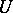ofis called a local chart ofwith domain of definition. Ifis endowed with the structure of a topological space, then it is further required thatbe an open subset ofand that the mappingbe a homeomorphism. A chart can similarly be defined with values in, where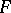is any normed field, and more generally, a chart can take values in a topological vector space. Two local charts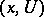,with domains of definitioninare said to be compatible of class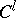if 1) their common domain of definition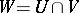is mapped by both charts onto an open set (that is, the sets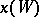and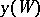are open in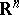); and 2) the coordinates of a point of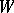with respect to one of these charts aretimes continuously-differentiable functions of the coordinates of the same point with respect to the other chart, that is, the vector function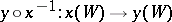istimes continuously differentiable. A family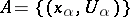of pairwise-compatible local chartsofthat cover(that is,) is called an atlas of. The specification of an atlas defines onthe structure of a differentiable manifold, and local charts that are compatible with all the charts of this atlas are said to be admissible (or-smooth).

The infinitesimal analogue of the notion of a chart is the concept of an infinitesimal chart of order(or a-jet (of a chart) or a co-frame of order). Two compatible local charts,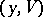of a setare said to be tangent to each other up to orderat a point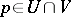if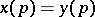and if all the partial derivatives up to order, inclusive, of the vector functionvanish at. The class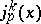of local charts tangent (up to order) at a point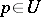of an admissible local chartof a differentiable manifoldis called the infinitesimal chart of orderat, or-jet at.

The choice of a chart on a manifoldallows one to consider various field quantities onas numerical functions and to apply to them the methods of analysis. In general, the value of a field quantity at a point depends on the choice of the chart. (Quantities which are independent of the choice of the chart are called scalars and are described by functions on.) However, for a wide and most important class of quantities (see Geometric objects, theory of), their value at a point depends only on the structure of the chart in the-th order infinitesimal neighbourhood of this point. Such quantities (examples of which are the tensor fields) are described by functions on the set of all co-frames of orderon. Along with these one studies the properties of quantities which do not depend on the choice of a chart. In this connection, the invariant coordinate-free approach to problems of differential geometry proves to be highly effective.

How to Cite This Entry:
Chart. Encyclopedia of Mathematics. URL: http://encyclopediaofmath.org/index.php?title=Chart&oldid=15612
This article was adapted from an original article by D.V. Alekseevskii (originator), which appeared in Encyclopedia of Mathematics - ISBN 1402006098. See original article